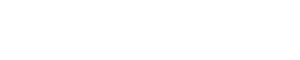# Strength of Materials

#### Course Topics

• INTRODUCTION
• ANALYSIS OF STRESES
• ANALYSIS OF STRAINS
• STRESS - STRAIN RELATIONS
• MEMBERS SUBJECTED TO UNIAXIAL STRESS
• THERMAL STRESSES
• CHANGE IN DIMENSIONS
• THIN SHELLS
• SHEAR & TORSION
• SPRINGS
• SHEAR FORCE & BENDING MOMENT
• PROCEDURES FOR DRAWING SHEAR FORCE & BENDING MOMENT
• SIMPLE BENDING THEORY
• USES OF FLEXURAL FORMULA
• SHEARING STRESS DISTRIBUTION IN TYPICAL CROSS SECTION
• DEFLECTION OF BEAMS
• MOMENT AREA METHOD
• MACAULAY'S METHOD
• COLUMN & STRUTS
• DEFINITIONS
• STRESS & STRAIN
• TRUE STRESS & TRUE STRAIN
• HOOKE'S LAW
• PRINCIPLES OF SUPERPOSITION
• MODULUS OF RESILIENCE
• STATISTICALLY INTERMEDIATE STRUCTURE
• RELATION BETWEEN YOUNG’S MODULUS, BULK MODULUS AND POISSON’S RATIO.
• POISSON’S RATIO
• Point Of Contraflexure’
• NEUTRAL AXIS IS A BEAM
• ASSUMPTIONS FOR FINDING OUT THE SHEAR STRESS IN A CIRCULAR SHAFT, SUBJECTED TO TORSION.
• SHEAR STRESS
• STRAIN ENERGY
• MODULUS OF SECTION FOR RECTANGULAR SECTION
• CIRCUMFERENTIAL STRESS AND LONGITUDINAL STRESS
• EXPRESSION FOR TORSIONAL SECTION MODULUS FOR SOLID CIRCULAR SHAFT
• STIFFNESS OF A CLOSED-COIL HELICAL SPRING
• LOAD FACTOR & IMPACT FACTOR
• POLAR MODULUZ
• HYDROSTATIC STATE OF STRESS
• TORSIONAL FLEXIBILITY AND TORSIONAL RIGIDITY
• YIELD POINT STRESS AND ULTIMATE STRESS.
• MODULUS OF RIGIDITY
• ELASTIC LIMIT AND PROPORTION LIMIT
• TOUGHNESS AND RESILIENCE
• FACTOR OF SAFETY
• STRAIN ENERGY
• PROOF RESILIENCE AND MODULUS OF RESILIENCE
• WIRE WINDING OF THIN CYLINDERS USES
• THIN CYLINDERS

#### INTRODUCTION

Engineering science is usually subdivided into number of topics such as

1.  Solid Mechanics

2.  Fluid Mechanics

3.  Heat Transfer

4.  Properties of materials and soon Although there are close links between them in terms of the physical principles involved and methods of analysis employed.

The solid mechanics as a subject may be defined as a branch of applied mechanics that deals with behaviours of solid bodies subjected to various types of loadings. This is usually subdivided into further two streams i.e Mechanics of rigid bodies or simply Mechanics and Mechanics of deformable solids.

The mechanics of deformable solids which is branch of applied mechanics is known by several names i.e. strength of materials, mechanics of materials etc.

Mechanics of rigid bodies:

The mechanics of rigid bodies is primarily concerned with the static and dynamic behaviour under external forces of engineering components and systems which are treated as infinitely strong and undeformable Primarily we deal here with the forces and motions associated with particles and rigid bodies.

Mechanics of deformable solids :

Mechanics of solids:

The mechanics of deformable solids is more concerned with the internal forces and associated changes in the geometry of the components involved. Of particular importance are the properties of the materials used, the strength of which will determine whether the components fail by breaking in service, and the stiffness of which will determine whether the amount of deformation they suffer is acceptable. Therefore, the subject of mechanics of materials or strength of materials is central to the whole activity of engineering design. Usually the objectives in analysis here will be the determination of the stresses, strains, and deflections produced by loads. Theoretical analyses and experimental results have an equal roles in this field.